Soliton Aided Propagation and Routing of Vortex Beams in Nonlocal Media | OMICS International
Journal of Lasers, Optics & Photonics

# Soliton Aided Propagation and Routing of Vortex Beams in Nonlocal Media

Gaetano Assanto1,2* and Noel F Smyth3

1NooEL- Nonlinear Optics and OptoElectronics Lab, University of Rome “Roma Tre”,00146 Rome, Italy

2The University of Wollongong, Wollongong, NSW 2522, australia

3School of Mathematics, University of Edinburgh, Edinburgh EH9 3JZ, Scotland, U.K

*Corresponding author:
Gaetano Assanto
Nonlinear Optics and Opto Electronics Lab
University of Rome “Roma Tre”, Italy
Tel: 39065733702
E-mail: [email protected]

Received Date: February 10, 2014; Accepted Date: April 22, 2014; Published Date: April 28, 2014

Citation: Assanto G, Smyth NF (2014) Soliton Aided Propagation and Routing of Vortex Beams in Nonlocal Media. J Laser Opt Photonics 1:105. doi:10.4172/2469-410X.1000105

Copyright: © 2014 Assanto G, et al. This is an open-access article distributed under the terms of the Creative Commons Attribution License, which permits unrestricted use, distribution, and reproduction in any medium, provided the original author and source are credited.

Visit for more related articles at Journal of Lasers, Optics & Photonics

#### Abstract

Two-dimensional optical vortex beams can propagate stably and undergo bending in nonlocal media with the aid of a spatial soliton, the latter preventing their destabilization and breakup. By colaunching a suitable pair of collinear soliton and vortex beams in nematic liquid crystals, we conduct a series of numerical experiments to demonstrate that the vortex beam can be made to propagate in the nematicon waveguide and follow its trajectory, even in the cases of refraction and total internal reflection at a dielectric interface. Modulation theory supports these findings and provides an excellent theoretical framework.

#### Keywords

Nematicons; Vortices; Solitons; Modulation Theory

#### Introduction

Finite light beams are subject to diffraction unless the latter is balanced by ADditional linear or nonlinear effects, e.g. by spatial dispersion or self-focusing or gain guiding [1-4]. In optically nonlinear media for which the refractive index increases with light excitation, twodimensional self-trapped beams take the form of spatial solitons and, in the presence of ADditional effects such as saturation or nonlocality or multiphoton absorption, can be stable and robust [5-8]. In the past fifteen years, optical spatial solitons in reorientational nonlocal media such as nematic liquid crystals have been among the most studied, both experimentally and theoretically/numerically [9,10]. The nonlocal index perturbation which supports the diffractionless propagation of a spatial soliton is, in fact, a grADed index waveguide able to guide light even of other wavelengths. The excitation, formation and control of nonlocal spatial solitons therefore links to the realization of versatile and reADdressable guided wave optical interconnects of potential impact in future generations of all-optical signal processors. More recently, beams with a complex phase and amplitude structure, such as vortices, have gained interest for particle manipulation and signal processing [11-15]. Linear vortices, however, naturally diffract and eventually loose their internal structure. Furthermore, intense vortex beams are subject to an azimuthal instability by which they tend to break up into two (or more) bright beams [16,17]. Therefore, the ability to stabilise and redirect vortex beams has become an important issue in beam optics, both in the linear and nonlinear regimes, particularly in nonlocal media. In the present work, with specific reference to nematic liquid crystals, but without loss of generality, we ADdress the stabilization and guidance of vortex beams by means of nonlocal bright spatial solitons, using modulation theory for and numerical solutions of the equations governing the nonlinear, nonlocal response of reorientational media. At variance with ``sombrero’’ refractive potentials, such as in antiguides , a bright soliton induces a dielectric waveguide and can, in some cases, confine and route a complex wavepacket such as a vortex. In this Paper we investigate collinear soliton-vortex pairs interacting with a dielectric interface and undergoing angular deviation by either refraction or total internal reflection. With the aid of a few specific numerical experiments for various wavepackets and a straight interface, we illustrate how a vortex can be soliton guided and deflected by the dielectric barrier, following the overall trajectory of the bright beam.

#### Geometry and Governing Equations

We consider a planar cell of finite uniform thickness filled with Nematic Liquid Crystals (NLC) into which two beams of copolarised light are injected and evolve in the midplane, parallel to the confining walls and without interacting with them. We take the propagation direction to be in (y,z) and the input polarisation direction of the light to be y, with x completing the coordinate triAD. For NLC aligned (by suitable anchoring at the interfaces) with the optic axis (molecular director n) in the plane (y,z), this launch geometry corresponds to light exciting the extraordinary eigenwaves in the uniaxial medium. At large enough light intensities, the optic axis can rotate in the (y,z) plane and thus increase the extraordinary refractive index, provided the electric field E and the director n are not mutually orthogonal, i.e. a torque can counteract the elastic intermolecular forces and minimize the system energy by rotating the light-induced dipoles towards the field vector . When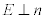, an external voltage bias can be applied across y to overcome the so-called Freédericksz threshold and set a finite initial angle θ0 of the elongated molecules to the E direction [9,20,21]. When E and n are coplanar at a finite non-zero angle <π/2, i.e. when the wavevector forms an angle 0< θ0 <π/2 with the optic axis, milliwatt light beams can rotate the NLC molecules by an extra amount θ, with |θ|=θ0, so that the total angle of the optic axis to z is θ0+θ and the refractive index for the extraordinarily polarized light increases accordingly and yields self-focusing . Since this reorientational response is nonresonant, refractive index changes can also be produced by voltage(s) applied across the NLC or by finite light beams travelling along y orthogonally to the solitary beam. Localized variations of the refractive index, in either one (interface) or two (defect) dimensions, can cause the beams propagating within the cell to refract, reflect, bend [9,22-31]. In the paraxial slowly varying envelope approximation, neglecting birefringent walkoff, the non-dimensional equations governing the evolution of extraordinarily polarised light beams of amplitudes u and v through the NLC are nonlinear Schrödinger (NLS)- type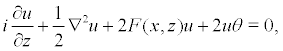(1)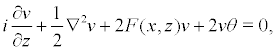(2)

for the wavepackets, and the elliptic equation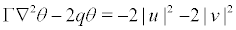(3)

for the reorientational response . Here u and v are the complex envelopes of the electric fields of the soliton (nematicon ) and vortex beams, respectively; F(x,z) indicates a y- uniform refractive index variation across the cell; the parameter Γ measures the elastic response of the medium and in the usual experimental regimes is large, 0(100) [9,33,34]; the parameter q is proportional to the magnitude of the external pre-tilting voltage (bias). In the present work the v beam carries an optical vortex, i.e. a finite two-dimensional wavepacket with a phase singularity (integer topological charge modulus 2π) on axis where its amplitude vanishes.

The equations (1)-(3) are studied hereby in the context of optical beams in reorientational nematic liquid crystals. This system of equations, however, is generic and applies to a wide range of physical situations, including nonlinear, nonlocal media for which the response to a beam involves some sort of diffusive mechanism , for instance thermo-optic media  such as leAD glasses [37-39] and photorefractive crystals . A similar system of equations also arises in the so-called α models of turbulence [41,42]. Moreover, while the system (1)-(3) is (2+1) dimensional as the z coordinate is time-like, the Schrödinger-Newton equations of quantum gravitation are of the same form, but three space dimensional .

In a planar NLC cell as described above we also consider a onedimensional refractive index barrier corresponding to a grADed index dielectric interface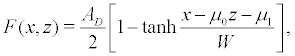(4)

as sketched in Figure 1 (left). This interface lies along the plane x=μ0z + μ1 and can yield an angular deviation of the wavepackets due to momentum (wavevector) conservation, i.e. upon refraction or Total Internal Reflection (TIR) as they travel from region 1 to region 2 (see Figure 1, right). In this expression, AD is the size of the index change across the interface and W is the width of the interface. The interface hAD to be smoothed from a discontinuous jump for the numerical solutions in order to prevent spurious, non-physical numerical effects such as beam splitting. The angular deviation of a nonlocal spatial soliton encountering an interface in NLC has been studied before, both experimentally and numerically [9,24, 44-48]. Here we focus on the propagation of a vortex beam which, colaunched with the soliton, is essentially guided by the latter and undergoes the same deflection without losing its complex phase and amplitude character.

Figure 1: (Color online) Left: Sketch of the nematic liquid crystal planar cell considered in this work, with dielectric regions 1 and 2 separated by a planar (y-invariant) grADed-index interface. Bell-shaped and vortex wavepackets are colaunched with collinear wavevectors at a finite angle of incidence to the interface. Right: refraction and total internal reflection of the beams reaching the interface from region 1.

We investigate the evolution of nematicon-vortex wavepackets upon refraction/TIR using both numerical solutions of the governing equations (1)-(3) and approximate evolution equations derived from modulation theory . The latter is based on a Lagrangian formulation of the governing equations. The Lagrangian for Equations. (1)-(3) is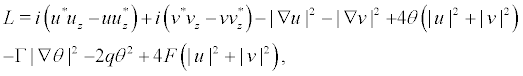(5)

where the superscript * denotes the complex conjugate.

Modulation theory is based on assuming appropriate trial functions for the evolving nematicon and vortex beams. Suitable trial functions are [10,50].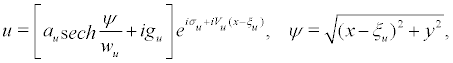(6)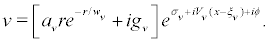(7)

Here (r,ϕ) are polar coordinates centred at (ξv,0) These functions consist of two parts: the first terms in each are a varying nematicon and a varying vortex, respectively; the second terms result from the low-wavenumber diffractive rADiation which accumulates under the wavepackets as they evolve due to its low group velocity . The parameters gu and gv measure the height of this shelf and do not depend on x and y. Since the rADiation cannot remain indefinitely flat, gu is non-zero in the disc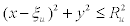centred on the nematicon  and gv is non-zero in the annulus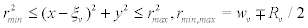located symmetrically about the peak of the vortex . The flat rADiation shelves under the beams then match to shed rADiation propagating away from them, enabling the beams to reach a steADy state [33,51,53]. The expressions for Ru and Rv=wv can be found in references  and , respectively.

The trial function for the molecular director deformation due to the light beams is more complicated. The director equation (3) is linear, so its solution can be broken up into the response due to the bright soliton and that due to the vortex. A suitable trial function for the director response to the soliton is .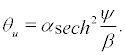(8)

While the vortex has zero amplitude at its core, the director distribution does not due to the nonlocality of NLC. A suitable trial function for the NLC response due to a vortex can be found by solving the director equation (3) in the limit of large nonlocality v [52,54] and is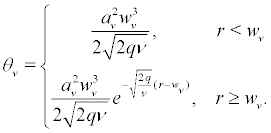(9)

The nonlocality produces an increase in θ (and extraordinary index) even in the vortex core (where the light amplitude vanishes), a phenomenon able to stabilise a nonlinear vortex in nematic liquid crystals [52,54]. The trial function for the molecular director is then θu + θv. All the parameters in the functions (6)-(9) depend on the evolution variable z.

The functions (6)-(9) are now substituted into the Lagrangian (5), which is then averaged by integrating in x and y from -∞ to ∞, resulting in the averaged Lagrangian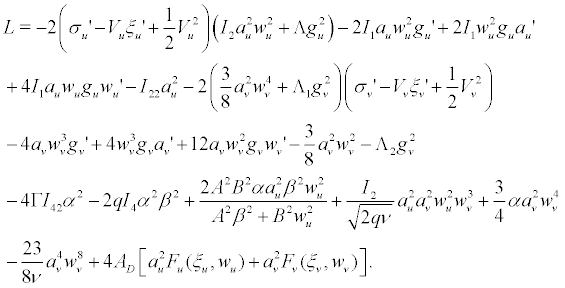(10)

The effects of the localised refractive index perturbation on the bright soliton and vortex beams are determined by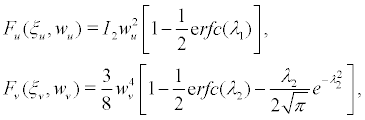(11)

respectively, where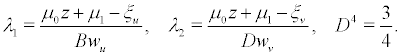(12)

The various integrals Ii and Iij in the averaged Lagrangian are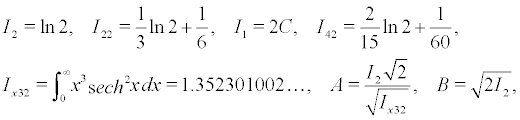(13)

where C is the Catalan constant C=0.915965594… . The terms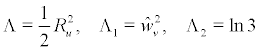(14)

arise due to the contributions of the shelves of diffractive rADiation under the wavepackets [50,52]. The superscript ^ denotes a steADy state value.

Taking variations of the averaged Lagrangian (10) with respect to the nematicon and vortex parameters results in the variational, or modulation , equations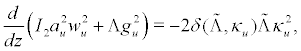(15)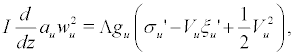(16)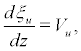(17)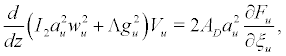(18)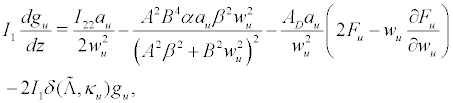(19)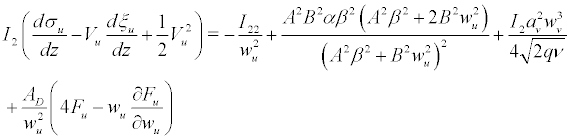(20)

for the spatial soliton and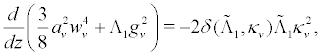(21)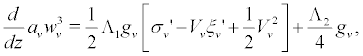(22)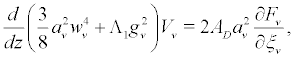(23)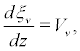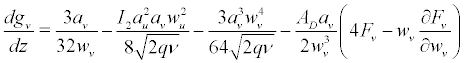(24)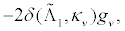(25)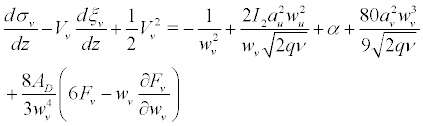(26)

for the vortex beam. The distribution of the molecular director is governed by the algebraic equations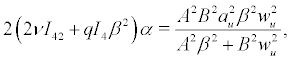(27)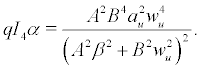(28)

Eliminating the director amplitude α between these equations gives the explicit solution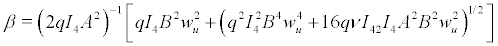(29)

for the width of the NLC response to the soliton.

The variational equations (15)-(26) have been augmented by the inclusion of losses due to shed diffractive rADiation. These loss terms are those with the parameter δ. This rADiative loss has been calculated elsewhere [50,53]. In the variational equations (15)-(26) the loss coefficient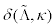is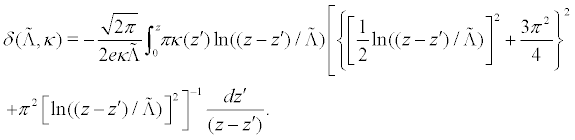(30)

Finally,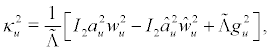(31)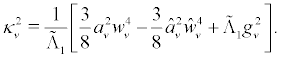(32)

where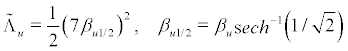(33)

and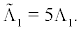(34)

In expressions (31) and (32) for κu and κv, the superscript ^ denotes fixed point values . There are not enough conservation laws to determine these steADy state values from the initial beams; hence, they are estimated using the modulation equations (19) and (25) to obtain the fixed point relations between the amplitude au and width wu of the nematicon and amplitude av and width wv of the vortex as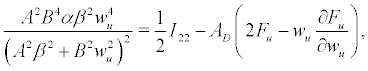(35)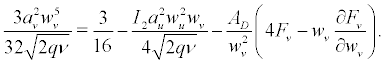(36)

Given values of wu and wv at z, these relations and the algebraic equations (27) and (29) are used to obtain

local approximations to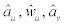and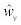yielding loss in good agreement with numerical solutions .

#### Results

Numerical solutions of the equations (1) and (2) were obtained using the pseudo-spectral method of Fornberg and Whitham  with the stepping forward in z performed in Fourier space using the 4th order Runge-Kutta method, rather than the leap-frog method in real space of Fornberg and Whitham. The elliptic director equation (3) was solved using a Fourier based method for elliptic equations . The numerical x position of the vortex was estimated by its centre of mass position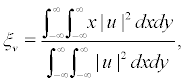(37)

as it moves in the (x,z) plane.

Refraction/TIR of a linear vortex

We consider the refraction of soliton guided vortices from a more to a less optically dense region, as this can potentially yield total internal reflection back to the region of incidence, as well as refraction. Vortex refraction from a rarer to a denser medium has been reported earlier .

Let us first study the interaction of a nematicon with a linear vortex beam of the form of a Laguerre-Gauss mode with zero rADial mode number, so that the initial wavepackets are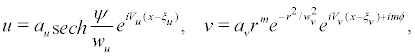(38)

where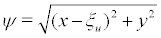and (r,ϕ) are plane polar coordinates centred at (ξv,0). The vortex charge m is a positive integer and quantifies the orbital angular momentum carried per photon in units of ħ. Here au and wu are the amplitude and width of the nematicon, av is related to the amplitude of the vortex and wv is its width. The nematicon is centred at (ξu,0) and has velocity Vu and the vortex has its singularity (centre) at (ξv,0) and velocity Vv. As the vortex is linear and does not affect the medium, the equations governing its interaction with the soliton are the electric field equations (1) and (2) and the director equation (3) with the |v|2 term neglected.

When travelling through an interface, a linear vortex beam can be refracted in a stable manner when guided by a nonlocal spatial soliton as the latter forms a potential well (waveguide) in which the vortex propagates . Nonlinear vortices in nematic liquid crystals can undergo stable refraction when propagating from a higher to a lower optical density region [60,61]. The TIR of a linear vortex guided by a soliton has not been investigated to date. Figure 2 shows an example of a linear vortex confined by a nonlocal soliton and reflected at an interface. Both the vortex and the nematicon were chosen to have low amplitude and large width, as a vortex becomes more stable as its width increases . It can be seen that the vortex is stable and is trapped within the potential well created by the soliton. Figure 2a and Figure 2b display the evolution of the nematicon and the vortex, respectively, in the (x,z) plane. The two wavepackets are somewhat distorted when in the vicinity of the interface, but reform as they propagate away from it. This distortion and re-formation is confirmed by the solutions at z=100 shown in Figure 2c - Figure 2d. The nematicon has been totally restored at z=100, but the vortex has shed a portion on interaction with the interface as it partially entered the region of lower refractive index upon TIR; this shedding can be seen in Figure 2b when the vortex is near the interface. The trajectories of the nematicon, as given by the location of its maximum, and the vortex, as given by its centre of mass position (37), are plotted in Figure 2e. The rapid jumps in the nematicon position are due to its distortion by the interface. Figure 2a shows that the nematicon develops two peaks in the proximity of the interface; since their amplitudes oscillate, sudden changes appear in the location of the maximum.

Figure 2: (Color online) Total internal reflection of a nematicon and a charge 1 linear vortex for the initial values au = 0.3, av = 0.15, wu = 7.0, wv = 8.0, Vu = Vv = 1.5, ξuv = 0, AD= -0.2, Г= 200, q=2, μ0 = 2.0, μ1 = -25, W = 2.0 and m = 1. (a) evolution of nematicon |u| in (x, z) plane, white line: interface, (b) evolution of linear vortex |v| in (x, z) plane, white line: interface, (c) nematicon |u| at z = 100, (d) linear vortex |v| at z = 100, (e) trajectories of nematicon (red, solid line) and vortex (green dot-dash line). Interface: blue dotted line.

Figure 3 displays the refraction of a guided linear vortex into the NLC region with lower index. In particular, Figure 3(d) shows that the linear vortex is completely stable upon refraction when guided by the nematicon, with the latter undergoing some distortion on interacting with the interface, which is most clearly seen from its maximum position in Figure 3e. The refraction of the two wavepackets illustrated in Figure 3a - Figure 3b resembles the refraction of a linear plane wave at a sharp index barrier, as governed by Snell’s Law.

Figure 3: (Color online) Refraction of a nematicon and a charge 1 linear vortex for the initial values au = 0.3, av = 0.15, wu = 7.0, wv = 8.0, Vu = Vv = 0.75, ξuv = 0, AD= -0.2, Γ= 200, q=2, μ0 = 2.0, μ1 = -25, W = 2.0 and m = 1. (a) evolution of nematicon |u| in (x, z) plane, white line: interface, (b) evolution of linear vortex |v| in (x, z) plane, white line: interface, (c) nematicon |u| at z = 60, (d) linear vortex |v| at z = 60, (e) trajectories of nematicon (red, solid line) and vortex (green dot-dash line). Interface: blue dotted line.

In contrast to the stable total internal reflection of a charge 1 linear vortex, the stable TIR of a charge 2 vortex was not achieved, with the vortex breaking up into two charge 1 vortices, as illustrated in Figure 4. It can be seen from Figure 4c that the nematicon has undergone stable TIR; in contrast, Figure 4d shows that the vortex has become unstable. As well as the splitting off of a portion of the vortex due to crossing the interface, as visible for the charge 1 vortex in Figure 2d, the charge 2 vortex has broken up into two charge 1 vortices, as indicated by the two nodes within the vortex in Figure 4d. The trajectories of the nematicon and vortex in Figure 4e indicate that both the soliton and the vortex beam have undergone TIR, even though in the process the charge 2 vortex has broken up into two charge 1 vortices.

Figure 4: (Color online) Total internal reflection of a nematicon and a charge 2 linear vortex for the initial values au = 0.25, av = 0.02, wu = 8.0, wv = 9.0, Vu = Vv = 1.5, ξuv = 0, AD= -0.2, Γ= 200, q=2, μ0 = 2.0, μ1 = -25, W = 2.0 and m = 2. (a) evolution of nematicon |u| in (x, z) plane, white line: interface, (b) evolution of linear vortex |v| in (x, z) plane,white line: interface, (c) nematicon |u| at z = 100, (d) linear vortex |v| at z = 100, (e) trajectories of nematicon (red, solid line) and vortex (green dotdash line). Interface: blue dotted line.

Refraction/TIR of a nonlinear vortex

Let us now consider the refraction and total internal reflection of a nonlinear optical vortex collinear with a nematicon. This evolution is governed by the full equations (1)-(3) and the modulation equations of Section 2, in particular (15)-(28).

Figure 5 is an example of the refraction of a nonlinear vortex at the interface. The latter was defined by a jump -0.1 in refractive index, reduced from the value (-0.2) used for the linear vortices in order to avoid the vortex splitting due to the azimuthal mode 2 instability [16,52]. The evolution of the nematicon and the vortex in the (x,z) plane, visible in Figure 5a and Figure 5b, respectively, is similar to that for the linear vortex, displayed in Figure 2a and Figure 2b. The main difference is that the amplitudes of the soliton and vortex have increased by a much larger amount upon refraction. This is due to the coupling between the nematicon and the nonlinear vortex, displayed in the modulation equations (15)-(28), particularly the coupling of their amplitudes au and av which can support bound states, i.e. nematicon-vortex vector solitons . The nematicon and vortex at z=60 are illustrated in Figure 5(c) and Figure 5(d). In particular, it can be seen that the vortex is stable upon refraction, due to the lower value of AD. Finally, Figure 5(e) shows excellent agreement between the trajectories of the nematicon and vortex as given by the full numerical and modulation solutions. The nematicon trajectories are in perfect agreement, with the vortex velocity as given by modulation theory slightly greater than that given by the numerical solution.

Figure 5: (Color online) Refraction of a nematicon and a charge 1 nonlinear vortex for the initial values au = 0.45, av = 0.25, wu = 5.0, wv = 5.5, Vu = Vv = 0.75, ξuv = 0, AD= -0.1, Γ= 200, q=2, μ0 = 2.0, μ1 = -25, W = 2.0 and m = 1. (a)evolution of nematicon |u| in (x, z) plane, white line: interface, (b) evolution of vortex |v| in (x, z) plane, white line: interface,(c) nematicon |u| at z = 60, (d) vortex |v| at z = 60, (e) numerical trajectories of nematicon (red, solid line) and vortex (green, dashed line), modulation trajectories of nematicon (black, dash-dash-dot line) and vortex (light blue, dot-dashed line). Interface: blue dotted line.

The increased nonlinear coupling between the nematicon and the nonlinear vortex, not withstanding their binding into a vector soliton and the accompanying amplitude enhancement upon refraction has major consequences for the total internal reflection of the guided vortex, as illustrated in Figure 6. Figures 6(a) and Figures 6(b) show the evolution of the nematicon and the vortex in the (x,z) plane, respectively. It can be seen from the evolution in Figure 6(b) that the vortex amplitude has more than doubled upon interaction with the interface, with a resulting decrease in width and the excitation of the mode 2 instability ; the nonlinear vortex breaks up into two nematicons, as seen from Figure 6(d) displaying the solution for the vortex at z=100. In contrast, the soliton solution in Figure 6(c) shows that the nematicon is stable upon TIR. This instability and breakup of a guided nonlinear vortex upon TIR is similar to that of a non-guided nonlinear vortex [60,61]. Since the modulation theory of Section 2 was based on the fixed beam profiles (6)-(9), as the vortex breaks up into two nematicons, good agreement is no longer expected between the numerical and modulation solutions in this unstable case. However, the trajectory comparisons of Figure 6e show that the nematicon trajectories as given by the numerical and modulation solutions are in excellent agreement. The vortex trajectories are in good agreement, unexpectedly; this is because the numerical vortex trajectory is given by the centre of mass position (37), and the agreement is due to total linear momentum being conserved by the vortex governed by Equation. (2).

Figure 6: (Color online) Total internal reflection of a nematicon and a charge 1 nonlinear vortex for the initial values au = 0.7, av = 0.1, wu = 5.0, wv = 6.0, Vu = Vv = 1.5, ξuv = 0, AD= -0.1, Γ= 200, q=2, μ0 = 2.0, μ1 = -25, W = 2.0 and m = 1. (a) evolution of nematicon |u| in (x, z) plane, white line: interface, (b) evolution of vortex |v| in (x, z) plane, white line: interface, (c) nematicon |u| at z = 100, (d) vortex |v| at z = 100, (e) calculated trajectories of nematicon (red, solid line) and vortex (green, dashed line), modulation trajectories of nematicon (black, dash-dash-dot line) and vortex (light blue, dot-dashed line). Interface: blue dotted line.

#### Conclusions

It was found that an optical solitary wave in nematic liquid crystals, a nematicon, can act as a waveguide for co-propagating linear or nonlinear optical vortices. This transverse confinement can stably guide linear and nonlinear vortices upon refraction at a dielectric interface. Despite stable trapping, however, total internal reflection of linear and nonlinear vortices travelling from an optically denser towards a rarer region is more involved. A linear vortex can undergo stable total internal reflection when guided by a nematicon. In contrast, we could not obtain the stable TIR of a nonlinear vortex as it exhibits the usual mode 2 instability  and breaks up into two bright solitons. This is due to a strong coupling between the nematicon and the nonlinear vortex which enhances its amplitude, resulting in instability.

Numerical experiments showed that a nematicon can guide a charge 1 linear vortex in a stable fashion upon both refraction and total internal reflection, but stable refraction and total internal reflection of a charge 2 vortex (or higher charge) was not achieved. While charge 1 vortices can be stabilised by the medium nonlocality, this response does not seem to stabilise higher charge vortices . Vortex beams do not possess the inherent stability and robustness of optical solitary waves, so these results show the utility of using nonlocal solitons to guide them along curved trajectories and provide guidelines for future experimental demonstrations of these phenomena in suitable media.

This work was supported by the Royal Society of London under grant IE111560. GA thanks Prof. T. Marchant and Prof. J. Xi for his fruitful visit at the University of Wollongong.

#### References

Select your language of interest to view the total content in your interested language

### Article Usage

• Total views: 12164
• [From(publication date):
June-2014 - Dec 05, 2019]
• Breakdown by view type
• HTML page views : 8371Can't read the image? click here to refresh